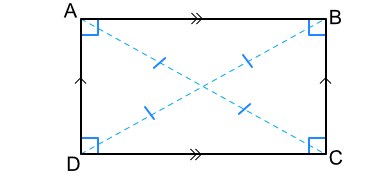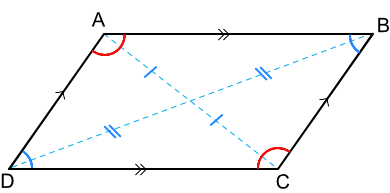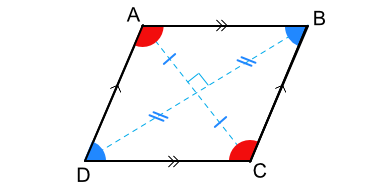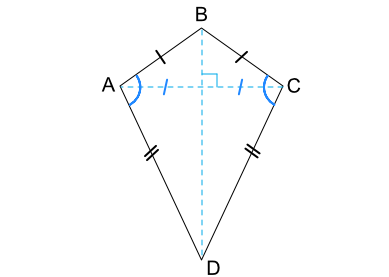# Properties of Quadrilaterals

GCSE(F),

Quadrilaterals are shapes with four sides.

In the following diagrams:
the arrows indicate parallel lines;
the marks on lines indicate lines that are bisected;
angles with the same value have the same colour;
filled angles are bisected by the diagonals.

Rectangle
All four interior angles are right anglesSquare
A rectangle with all sides of equal lengthParallelogram
Two sets of parallel sidesRhombus
A parallelogram with equal length sidesKite
A quadrilateral reflected in the line BDTrapezium
One set of parallel sides
DAB + CDA = 180º
ABC + BCD = 180ºtrapezium properties

## Examples

1. Give one difference between a trapezium and a parallelogram.

Answer: A parallelogram has two sets of parallel sides: a trapezium only has one set

A parallelogram has two sets of parallel sides: a trapezium only has one set

2. What is the size of the angle CDA in this kite?>